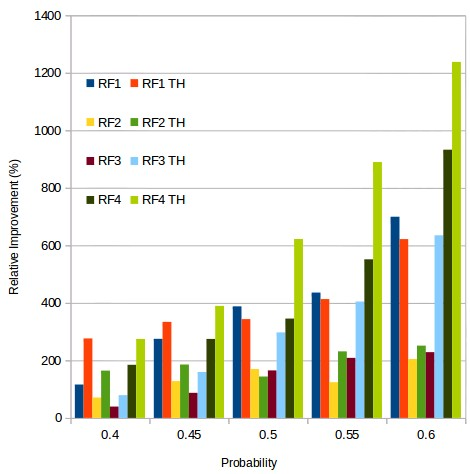## When are non-zero return thresholds a good idea for OS performance classification?

In my previous post we discussed the use of return thresholds in the creation of a classifier in order to improve the out-of-sample (OS) performance of trading strategies. In essence instead of simply predicting whether a system’s future return was above or below zero we tried to predict whether the return was above or below a given threshold (Th). This showed to significantly increase relative performance within testing sets for a sample random forest (RF) machine learning algorithm for OS result classification.  However – after trying on a few other algorithms – it became clear that the idea of using a non-zero return threshold for classification does not work effectively across all OS classifiers. In today’s post I want to discuss these results and when it might be a good or bad idea to apply this technique.The entire idea of predicting a non-zero return instead of a zero return comes from a desire to eliminate marginal decision making. When you use the results of an OS classifier for trading a strategy you might want to avoid trading not only when the algorithm predicts you will have a negative return, but you might even want to avoid getting into the market unless the predicted reward is high enough. In order to achieve this effect the simplest thing to do is to change the classification threshold to our “minimum desired average return” and then train our OS classifier to predict whether or not we will be able to obtain a result that is above this point. If our classifier then tells us that we have a higher than 50% probability to obtain a result above this return then we should enter the market to take advantage of this opportunity.

This sounds really good and showed very good improvements in results in my previous post. However – when looking at other algorithms – it became clear that this is not always the case. The picture above shows you the relative improvement in results for 4 different random forest classifiers that use different types of inputs and windows for predictions, you can see the results when using zero as a threshold (RFX) or when using a Th value of 0.05% for classification (RFX TH) at different probability thresholds. The results show that for the RF1 and RF2 algorithms the introduction of the non-zero Th for classification actually makes results worse, almost regardless of the actual probability threshold used to make the trading decision. This is in great contrast to algorithms RF3 and RF4 where the introduction of this probability threshold increases the improvement in results dramatically, even taking us above the 1000% mark for the RF4 TH when making the decision to trade only above a 0.6 probability.But why is this the case? The 4 different algorithms aren’t extremely different in the quality or number of inputs they use. It is true that RF3 and RF4 do use more inputs, but the actual improvements in results obtained at the zero Th level is actually similar for RF1, RF2 and RF3, although for RF3 there is a huge increase in profitability when using the Th of 0.05% that doesn’t really exist for the RF1 or RF2 algorithms. Looking at the second plot in this post, which shows how the sensitivity changes for the 4 different algorithms, we start to see some hints about the real reasons that might make the use of a non-zero Th better in some cases than in others.

I want you to take a look at the difference in sensitivity between the zero and non-zero Th results and especially the difference in sensitivity between both cases. The sensitivity drops as a function of the forecasting probability in all cases – as is expected – but in the case of the RF1 and RF2 algorithms the drop in sensitivity is such that when we go from zero to non-zero Th the sensitivity does not have a lot of way to drop beyond where it has already gone. This tells us that the classifiers in RF1 and RF2 are already having trouble getting true positives at a zero threshold and increasing the Th to a non-zero value does not actually help the classifier since the classifier does not even understand that well how to make a zero-based classification effectively. In contrast the sensitivity for the zero case is much higher at high forecasting probability thresholds for the zero Th value for RF3 and RF4, showing us that the classifier has a much better idea of how to discern strategies using the information at hand and therefore it can be pushed further. It can benefit from a non-zero Th.Ultimately this boils down to a matter of how much useful information the classifier is actually being able to gather, which is reflected in the sensitivity. A classifier that is able to have a slower drop in sensitivity as a function of its forecasting probability has a higher amount of “domain knowledge” and is therefore able to apply it to more stringent analysis. In all my tests algorithms that have benefited from a higher classification Th have always had sensitivities of at least 20% at a forecasting probability of 0.6 when using a zero Th value. This shows that the speed of the decline of sensitivity as a function of forecasting probability is a good tool to evaluate whether or not a non-zero Th for classification makes sense. If you would like to learn more about our machine learning algorithms for system selection and how you too can trade our system repository using this type of ML derived insights please consider joining Asirikuy.com, a website filled with educational videos, trading systems, development and a sound, honest and transparent approach towards automated trading.

You can leave a response, or trackback from your own site.